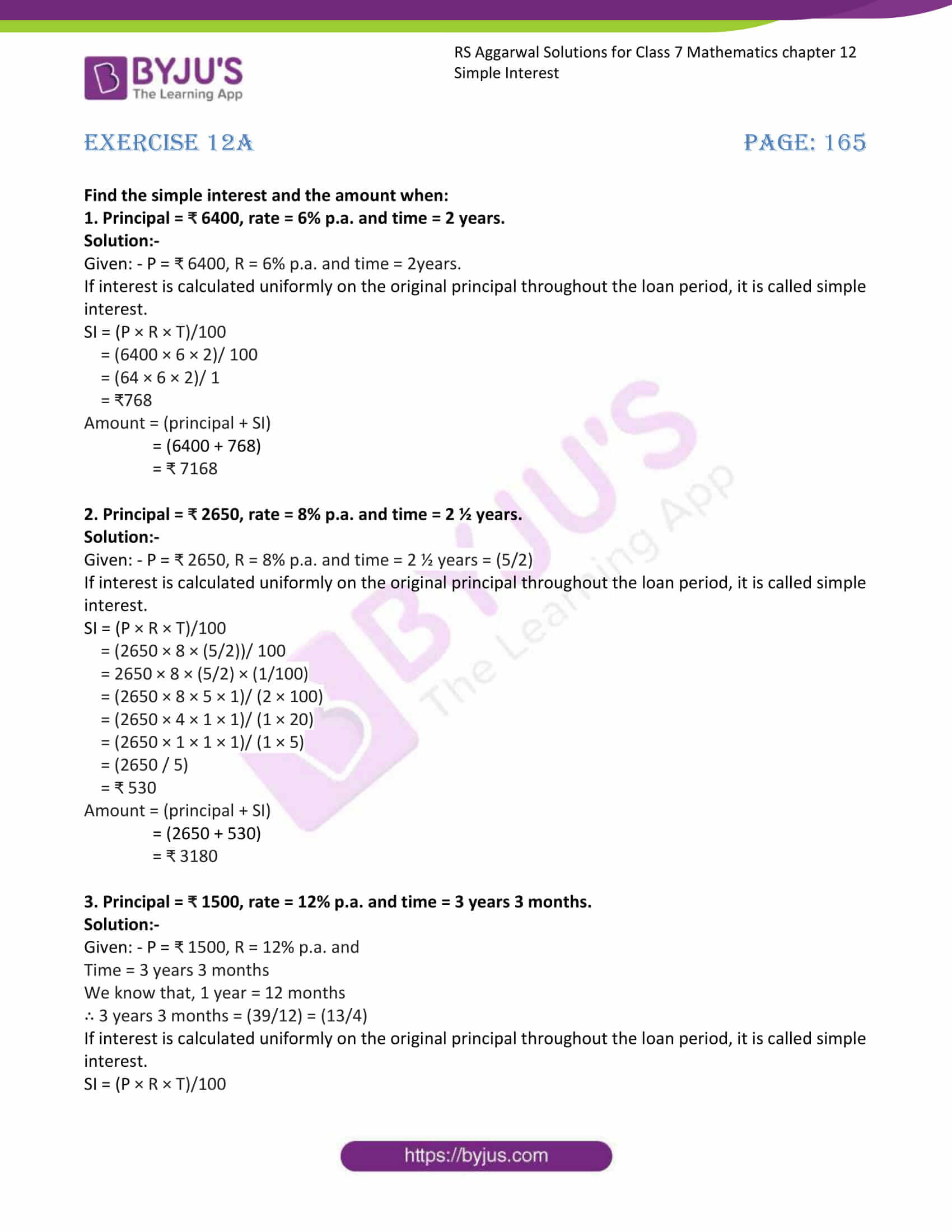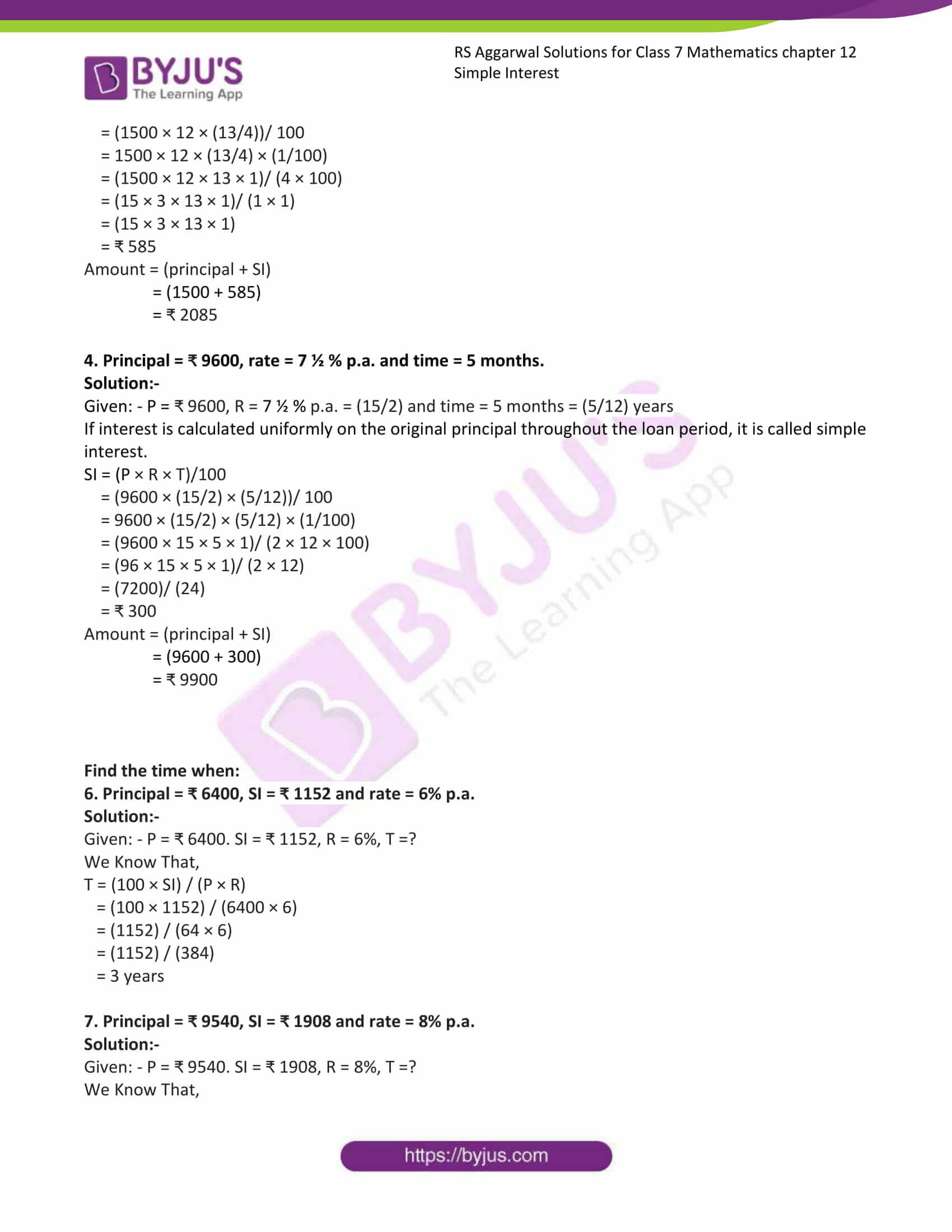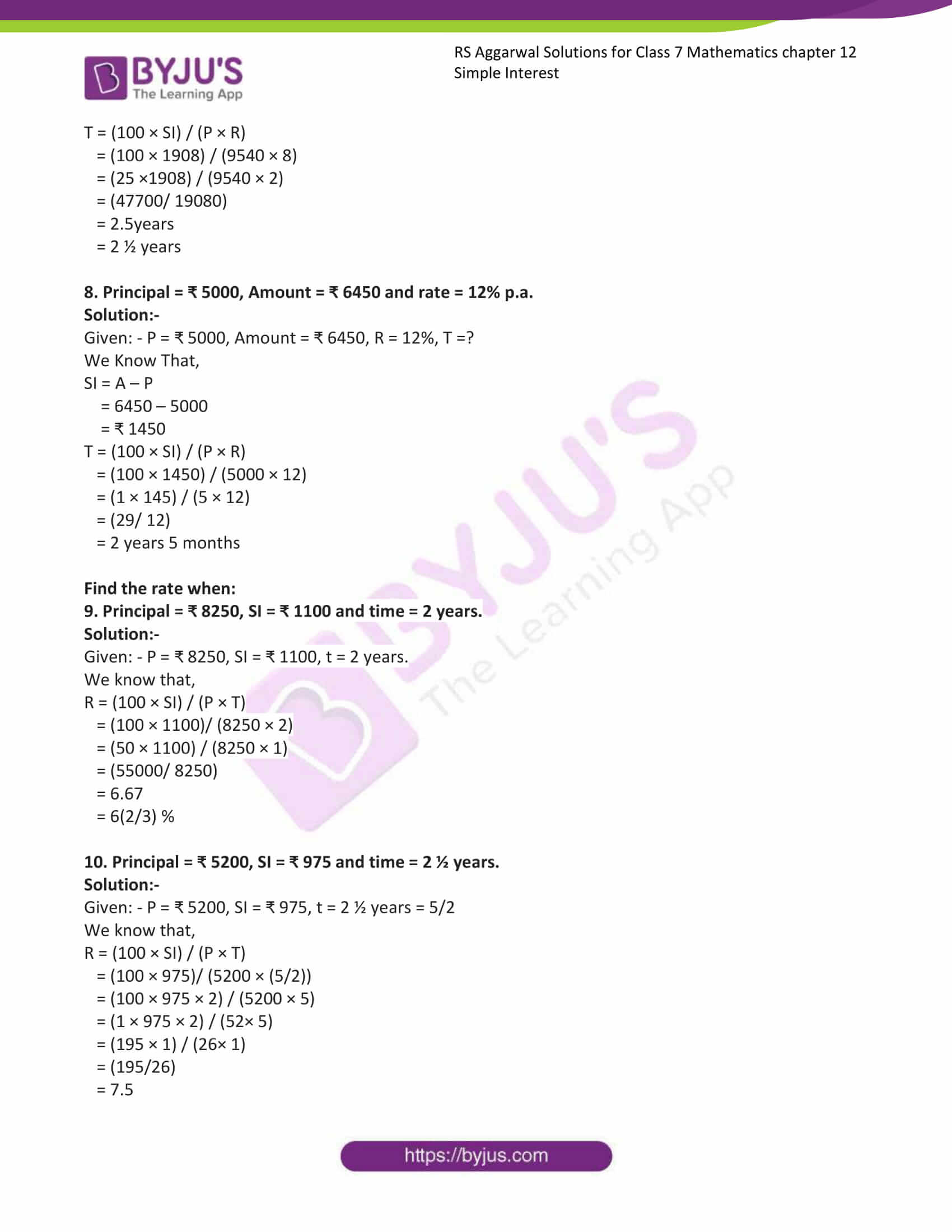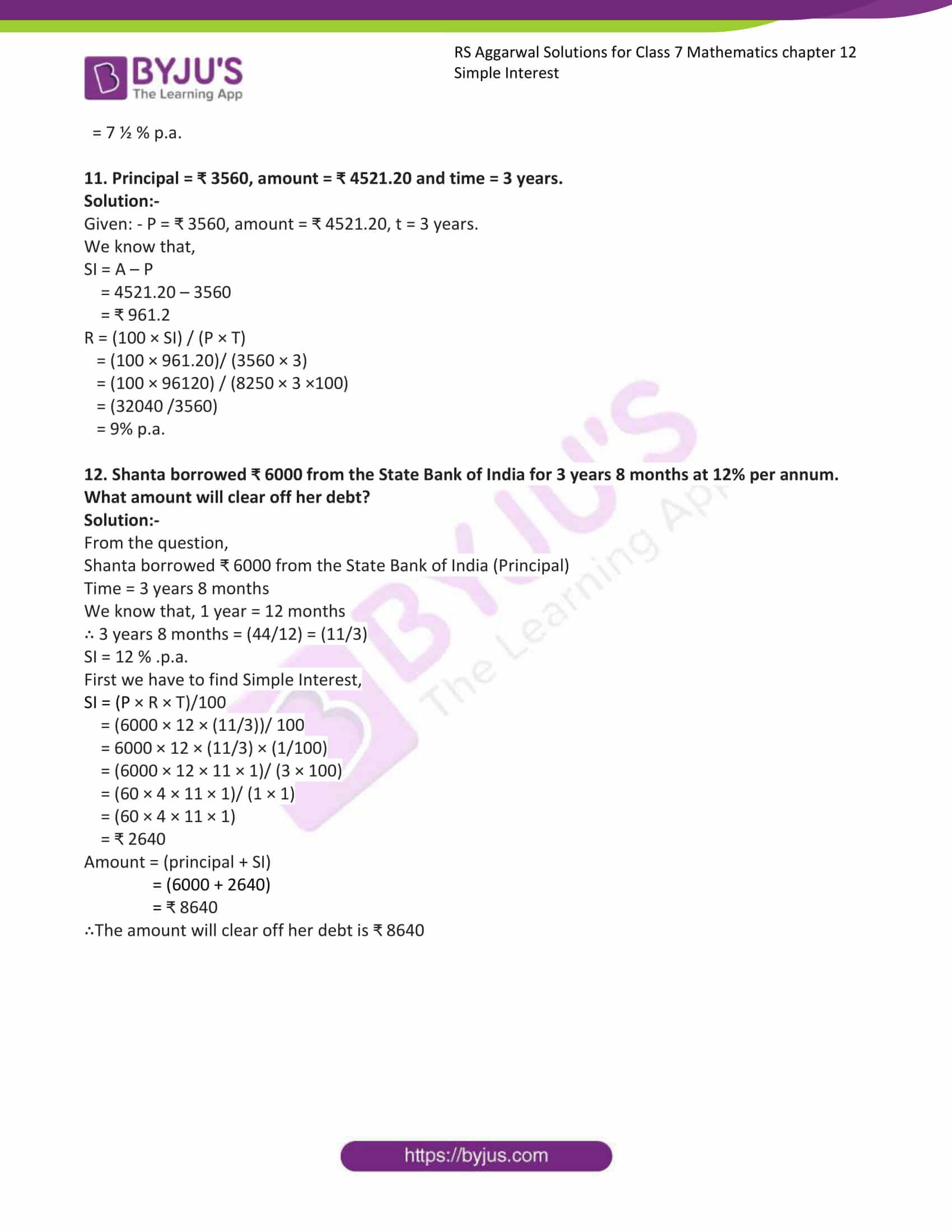# RS Aggarwal Solutions for Class 7 Maths Exercise 12A Chapter 12 Simple Interest

RS Aggarwal Solutions for Class 7 Maths Exercise 12A Chapter 12 Simple Interest in PDF are given here. We advise students to go through this RS Aggarwal Solutions for Class 7 Maths Chapter 12 Simple Interest to get good marks in board exams. Sometimes we need to borrow money from a bank or a moneylender for a specified period of time. At the end of the period, we have to pay back the money which we had borrowed plus some additional money for using the lender’s money. The topics covered in this exercise are principal, interest, amount and rate. Then students can score good marks in Maths by practising RS Aggarwal Class 7 Solutions.

## Download the PDF of RS Aggarwal Solutions For Class 7 Maths Chapter 12 Simple Interest – Exercise 12A### Access answers to Maths RS Aggarwal Solutions for Class 7 Chapter 12 – Simple Interest 12A

Find the simple interest and the amount when:

1. Principal = ₹ 6400, rate = 6% p.a. and time = 2 years.

Solution:-

Given: – P = ₹ 6400, R = 6% p.a. and time = 2years.

If interest is calculated uniformly on the original principal throughout the loan period, it is called simple interest.

SI = (P × R × T)/100

= (6400 × 6 × 2)/ 100

= (64 × 6 × 2)/ 1

= ₹768

Amount = (principal + SI)

= (6400 + 768)

= ₹ 7168

2. Principal = ₹ 2650, rate = 8% p.a. and time = 2 ½ years.

Solution:-

Given: – P = ₹ 2650, R = 8% p.a. and time = 2 ½ years = (5/2)

If interest is calculated uniformly on the original principal throughout the loan period, it is called simple interest.

SI = (P × R × T)/100

= (2650 × 8 × (5/2))/ 100

= 2650 × 8 × (5/2) × (1/100)

= (2650 × 8 × 5 × 1)/ (2 × 100)

= (2650 × 4 × 1 × 1)/ (1 × 20)

= (2650 × 1 × 1 × 1)/ (1 × 5)

= (2650 / 5)

= ₹ 530

Amount = (principal + SI)

= (2650 + 530)

= ₹ 3180

3. Principal = ₹ 1500, rate = 12% p.a. and time = 3 years 3 months.

Solution:-

Given: – P = ₹ 1500, R = 12% p.a. and

Time = 3 years 3 months

We know that, 1 year = 12 months

∴ 3 years 3 months = (39/12) = (13/4)

If interest is calculated uniformly on the original principal throughout the loan period, it is called simple interest.

SI = (P × R × T)/100

= (1500 × 12 × (13/4))/ 100

= 1500 × 12 × (13/4) × (1/100)

= (1500 × 12 × 13 × 1)/ (4 × 100)

= (15 × 3 × 13 × 1)/ (1 × 1)

= (15 × 3 × 13 × 1)

= ₹ 585

Amount = (principal + SI)

= (1500 + 585)

= ₹ 2085

4. Principal = ₹ 9600, rate = 7 ½ % p.a. and time = 5 months.

Solution:-

Given: – P = ₹ 9600, R = 7 ½ % p.a. = (15/2) and time = 5 months = (5/12) years

If interest is calculated uniformly on the original principal throughout the loan period, it is called simple interest.

SI = (P × R × T)/100

= (9600 × (15/2) × (5/12))/ 100

= 9600 × (15/2) × (5/12) × (1/100)

= (9600 × 15 × 5 × 1)/ (2 × 12 × 100)

= (96 × 15 × 5 × 1)/ (2 × 12)

= (7200)/ (24)

= ₹ 300

Amount = (principal + SI)

= (9600 + 300)

= ₹ 9900

Find the time when:

6. Principal = ₹ 6400, SI = ₹ 1152 and rate = 6% p.a.

Solution:-

Given: – P = ₹ 6400. SI = ₹ 1152, R = 6%, T =?

We Know That,

T = (100 × SI) / (P × R)

= (100 × 1152) / (6400 × 6)

= (1152) / (64 × 6)

= (1152) / (384)

= 3 years

7. Principal = ₹ 9540, SI = ₹ 1908 and rate = 8% p.a.

Solution:-

Given: – P = ₹ 9540. SI = ₹ 1908, R = 8%, T =?

We Know That,

T = (100 × SI) / (P × R)

= (100 × 1908) / (9540 × 8)

= (25 ×1908) / (9540 × 2)

= (47700/ 19080)

= 2.5years

= 2 ½ years

8. Principal = ₹ 5000, Amount = ₹ 6450 and rate = 12% p.a.

Solution:-

Given: – P = ₹ 5000, Amount = ₹ 6450, R = 12%, T =?

We Know That,

SI = A – P

= 6450 – 5000

= ₹ 1450

T = (100 × SI) / (P × R)

= (100 × 1450) / (5000 × 12)

= (1 × 145) / (5 × 12)

= (29/ 12)

= 2 years 5 months

Find the rate when:

9. Principal = ₹ 8250, SI = ₹ 1100 and time = 2 years.

Solution:-

Given: – P = ₹ 8250, SI = ₹ 1100, t = 2 years.

We know that,

R = (100 × SI) / (P × T)

= (100 × 1100)/ (8250 × 2)

= (50 × 1100) / (8250 × 1)

= (55000/ 8250)

= 6.67

= 6(2/3) %

10. Principal = ₹ 5200, SI = ₹ 975 and time = 2 ½ years.

Solution:-

Given: – P = ₹ 5200, SI = ₹ 975, t = 2 ½ years = 5/2

We know that,

R = (100 × SI) / (P × T)

= (100 × 975)/ (5200 × (5/2))

= (100 × 975 × 2) / (5200 × 5)

= (1 × 975 × 2) / (52× 5)

= (195 × 1) / (26× 1)

= (195/26)

= 7.5

= 7 ½ % p.a.

11. Principal = ₹ 3560, amount = ₹ 4521.20 and time = 3 years.

Solution:-

Given: – P = ₹ 3560, amount = ₹ 4521.20, t = 3 years.

We know that,

SI = A – P

= 4521.20 – 3560

= ₹ 961.2

R = (100 × SI) / (P × T)

= (100 × 961.20)/ (3560 × 3)

= (100 × 96120) / (8250 × 3 ×100)

= (32040 /3560)

= 9% p.a.

12. Shanta borrowed ₹ 6000 from the State Bank of India for 3 years 8 months at 12% per annum. What amount will clear off her debt?

Solution:-

From the question,

Shanta borrowed ₹ 6000 from the State Bank of India (Principal)

Time = 3 years 8 months

We know that, 1 year = 12 months

∴ 3 years 8 months = (44/12) = (11/3)

SI = 12 % .p.a.

First we have to find Simple Interest,

SI = (P × R × T)/100

= (6000 × 12 × (11/3))/ 100

= 6000 × 12 × (11/3) × (1/100)

= (6000 × 12 × 11 × 1)/ (3 × 100)

= (60 × 4 × 11 × 1)/ (1 × 1)

= (60 × 4 × 11 × 1)

= ₹ 2640

Amount = (principal + SI)

= (6000 + 2640)

= ₹ 8640

∴The amount will clear off her debt is ₹ 8640

### Access other exercises of RS Aggarwal Solutions For Class 7 Chapter 12 – Simple Interest

Exercise 12B Solutions